# JP5211263B2 - Method and apparatus for local multiple hypothesis prediction during video coding of coding unit - Google Patents

Method and apparatus for local multiple hypothesis prediction during video coding of coding unit Download PDF

## Info

Publication number
JP5211263B2
JP5211263B2 JP2012510110A JP2012510110A JP5211263B2 JP 5211263 B2 JP5211263 B2 JP 5211263B2 JP 2012510110 A JP2012510110 A JP 2012510110A JP 2012510110 A JP2012510110 A JP 2012510110A JP 5211263 B2 JP5211263 B2 JP 5211263B2
Authority
JP
Japan
Prior art keywords
unit
sub
encoding
motion
encoding unit
Prior art date
Legal status (The legal status is an assumption and is not a legal conclusion. Google has not performed a legal analysis and makes no representation as to the accuracy of the status listed.)
Active
Application number
JP2012510110A
Other languages
Japanese (ja)
Other versions
JP2012513179A (en
Inventor
リン，ジエン−リィアン
ツァイ，ユー−バオ
レイ，シャオ−ミン
Original Assignee
メディアテック インコーポレーテッドＭｅｄｉａｔｅｋ Ｉｎｃ．
Priority date (The priority date is an assumption and is not a legal conclusion. Google has not performed a legal analysis and makes no representation as to the accuracy of the date listed.)
Filing date
Publication date
Priority to US32394810P priority Critical
Priority to US61/323,948 priority
Priority to US13/080,668 priority patent/US8879620B2/en
Priority to US13/080,668 priority
Application filed by メディアテック インコーポレーテッドＭｅｄｉａｔｅｋ Ｉｎｃ． filed Critical メディアテック インコーポレーテッドＭｅｄｉａｔｅｋ Ｉｎｃ．
Priority to PCT/CN2011/072783 priority patent/WO2011127828A1/en
Publication of JP2012513179A publication Critical patent/JP2012513179A/en
Application granted granted Critical
Publication of JP5211263B2 publication Critical patent/JP5211263B2/en
Active legal-status Critical Current
Anticipated expiration legal-status Critical

• 238000000034 methods Methods 0.000 claims description 35
• 230000000875 corresponding Effects 0.000 claims description 22
• 230000002123 temporal effects Effects 0.000 claims description 20
• 238000001914 filtration Methods 0.000 claims description 5
• 238000005457 optimization Methods 0.000 claims description 4
• 230000004048 modification Effects 0.000 description 41
• 238000006011 modification reactions Methods 0.000 description 41
• 238000010586 diagrams Methods 0.000 description 20
• 238000007781 pre-processing Methods 0.000 description 8
• 239000010949 copper Substances 0.000 description 7
• 241000190080 Turneraceae Species 0.000 description 5
• 238000005192 partition Methods 0.000 description 5
• 230000037010 Beta Effects 0.000 description 3
• 208000003960 Microvillus inclusion diseases Diseases 0.000 description 2
• 239000000969 carriers Substances 0.000 description 2
• 238000007906 compression Methods 0.000 description 2
• 230000014509 gene expression Effects 0.000 description 2
• 230000000903 blocking Effects 0.000 description 1
• 238000010187 selection method Methods 0.000 description 1
• 238000000638 solvent extraction Methods 0.000 description 1

## Images

•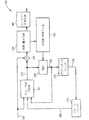•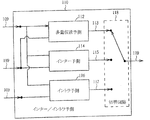•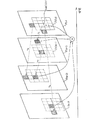•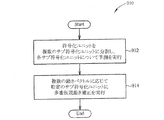•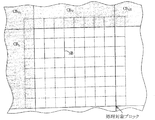•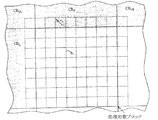•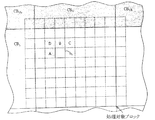•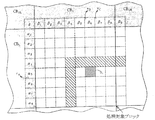•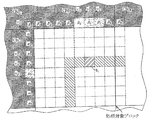•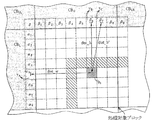•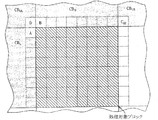•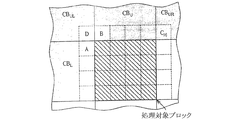•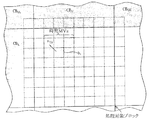•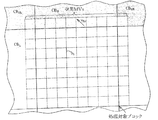•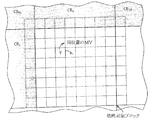•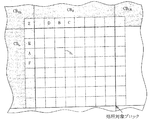•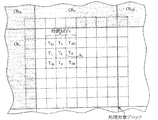•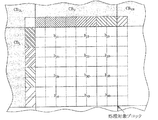•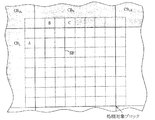•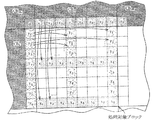## Classifications

• HELECTRICITY
• H04ELECTRIC COMMUNICATION TECHNIQUE
• H04NPICTORIAL COMMUNICATION, e.g. TELEVISION
• H04N19/00Methods or arrangements for coding, decoding, compressing or decompressing digital video signals
• H04N19/50Methods or arrangements for coding, decoding, compressing or decompressing digital video signals using predictive coding
• H04N19/503Methods or arrangements for coding, decoding, compressing or decompressing digital video signals using predictive coding involving temporal prediction
• H04N19/51Motion estimation or motion compensation
• H04N19/56Motion estimation with initialisation of the vector search, e.g. estimating a good candidate to initiate a search
• HELECTRICITY
• H04ELECTRIC COMMUNICATION TECHNIQUE
• H04NPICTORIAL COMMUNICATION, e.g. TELEVISION
• H04N19/00Methods or arrangements for coding, decoding, compressing or decompressing digital video signals
• H04N19/10Methods or arrangements for coding, decoding, compressing or decompressing digital video signals using adaptive coding
• H04N19/134Methods or arrangements for coding, decoding, compressing or decompressing digital video signals using adaptive coding characterised by the element, parameter or criterion affecting or controlling the adaptive coding
• H04N19/136Incoming video signal characteristics or properties
• H04N19/137Motion inside a coding unit, e.g. average field, frame or block difference
• H04N19/139Analysis of motion vectors, e.g. their magnitude, direction, variance or reliability
• HELECTRICITY
• H04ELECTRIC COMMUNICATION TECHNIQUE
• H04NPICTORIAL COMMUNICATION, e.g. TELEVISION
• H04N19/00Methods or arrangements for coding, decoding, compressing or decompressing digital video signals
• H04N19/50Methods or arrangements for coding, decoding, compressing or decompressing digital video signals using predictive coding
• H04N19/503Methods or arrangements for coding, decoding, compressing or decompressing digital video signals using predictive coding involving temporal prediction
• H04N19/51Motion estimation or motion compensation
• HELECTRICITY
• H04ELECTRIC COMMUNICATION TECHNIQUE
• H04NPICTORIAL COMMUNICATION, e.g. TELEVISION
• H04N19/00Methods or arrangements for coding, decoding, compressing or decompressing digital video signals
• H04N19/50Methods or arrangements for coding, decoding, compressing or decompressing digital video signals using predictive coding
• H04N19/503Methods or arrangements for coding, decoding, compressing or decompressing digital video signals using predictive coding involving temporal prediction
• H04N19/51Motion estimation or motion compensation
• H04N19/513Processing of motion vectors
• HELECTRICITY
• H04ELECTRIC COMMUNICATION TECHNIQUE
• H04NPICTORIAL COMMUNICATION, e.g. TELEVISION
• H04N19/00Methods or arrangements for coding, decoding, compressing or decompressing digital video signals
• H04N19/50Methods or arrangements for coding, decoding, compressing or decompressing digital video signals using predictive coding
• H04N19/503Methods or arrangements for coding, decoding, compressing or decompressing digital video signals using predictive coding involving temporal prediction
• H04N19/51Motion estimation or motion compensation
• H04N19/513Processing of motion vectors
• H04N19/517Processing of motion vectors by encoding
• H04N19/52Processing of motion vectors by encoding by predictive encoding

## Description

Detailed Description of the Invention

[Cross-reference of related applications]
This application is filed in US provisional application no. 61 / 323,948 (filed on April 14, 2010, title of invention: multiple hypothesis prediction in video coding), and claims the priority, and includes the contents described in the application. In addition, this application is a US application no. No. 13/080668 (filed on Apr. 6, 2011, title of invention: method and apparatus for performing local multiple hypothesis prediction at the time of video coding of a coding unit), and claims the priority. The contents are included.

〔Technical field〕
The present invention relates to video motion compensation. More specifically, the present invention relates to a method and apparatus for performing localized multihypothesis prediction when encoding an image of an encoding unit.

[Background Technology]
Motion compensation is a technique used in encoding and decoding video data for video compression and decompression. For motion compensation, the current image is represented by partially replacing one or more reference images. The reference image may be temporally before the current time or may be temporally after the current time. Typically, when motion compensation is used, the image is precisely synthesized with a previously transmitted or stored image to improve compression efficiency. With the advancement of coding technology, a new standard design was introduced. However, in the new standard, the conventional motion compensation scheme does not always work properly. For example, the encoding efficiency may decrease. For this reason, a new method for improving the coding efficiency of video coding is required.

[Summary of the Invention]
The present invention has been made to solve the above problems, and an object of the present invention is to provide a method and an apparatus for performing local multiple hypothesis prediction at the time of video coding of a coding unit.

An exemplary embodiment of the present invention that performs local multiple hypothesis prediction during video encoding of an encoding unit includes a dividing step of dividing the encoding unit into a plurality of sub encoding units, and each of the sub encoding units. Processing steps to be processed. More specifically, the processing step includes obtaining a first set of motion information of an encoded unit for multiple hypothesis motion compensation of a specific sub-encoding unit of the sub-encoding units; A use step of using a linear combination of a plurality of pixel values obtained from the first set of motion information of the encoded unit as a predicted pixel value of the specific sub-encoding unit; and included in the first set of encoded units And calculating a predicted pixel value of another sub-encoding unit in the encoding unit using a second set of motion information of the encoded unit including at least one encoded unit.

An exemplary embodiment of the present invention for an apparatus that performs local multiple hypothesis prediction during video encoding of an encoding unit includes a processing circuit that performs video encoding in the encoding unit, and the processing circuit includes: Includes a pre-processing section. The preliminary processing unit divides the encoding unit into a plurality of sub-encoding units, and performs processing for each sub-encoding unit. More specifically, the preliminary processing unit obtains motion information of the first set of encoded units to perform multiple hypothesis motion compensation processing on a specific sub-encoding unit among the plurality of sub-encoding units. And applying multiple hypothesis motion compensation to the specific sub-encoding unit in accordance with the motion information, and linearly combining a plurality of pixel values obtained from the first set of motion information of the encoded unit. Used as the predicted pixel value of the unit. Further, the preliminary processing unit uses the motion information of the second set of the encoded unit including at least one encoding unit that is not included in the first set of encoded units, Predictive pixel values of the sub-encoding units are calculated.

In addition, another exemplary embodiment of the present invention that performs local multiple hypothesis prediction at the time of video encoding of an encoding unit includes a dividing step of dividing the encoding unit into a plurality of sub encoding units, Processing steps for processing the encoding unit. More specifically, the processing step includes an acquisition step of acquiring motion information of a plurality of encoded units for multiple hypothesis motion compensation of a specific sub-encoding unit of the sub-encoding units, and the code A use step of using a weighted sum of a plurality of pixel values obtained from the motion information of the encoded unit as a predicted pixel value of the specific sub-encoding unit, and a pixel value of the pixel value obtained from the motion information of the encoded unit. And calculating a predicted pixel value of another sub-encoding unit in the encoding unit by using another weighted sum.

The above objects and other objects of the present invention will become apparent to those skilled in the art from the following detailed description of the illustrated preferred embodiment.

[Brief description of the drawings]
FIG. 1A is a diagram illustrating an apparatus for performing local multiple hypothesis prediction during video coding of a coding unit according to an embodiment of the present invention.

1B is a diagram showing an inter / intra prediction unit provided in the apparatus shown in FIG. 1A.

FIG. 1C is a diagram showing a multiple hypothesis motion compensation process of the multiple hypothesis inter prediction circuit shown in FIG. 1B.

FIG. 2 is a flowchart of a method for performing local multiple hypothesis prediction during video coding of a coding unit according to an embodiment of the present invention.

FIG. 3 is a diagram illustrating an example of an encoded block in the method illustrated in FIG.

FIG. 4 is a diagram showing details of processing performed in the method shown in FIG.

5A to 5D are diagrams showing details of multiple hypothesis prediction performed in the method shown in FIG.

6A to 6B are diagrams illustrating a motion information acquisition process performed in the method illustrated in FIG.

7A to 7B are diagrams showing temporal motion vectors MVs and spatial motion vectors MVs in the method shown in FIG. 2, respectively.

8A to 8C are diagrams illustrating a motion vector acquisition process performed in the method illustrated in FIG.

FIG. 9 is a diagram showing processing performed in the method shown in FIG.

FIG. 10 is a diagram showing processing performed in the method shown in FIG.

FIG. 11 is a diagram showing processing performed in the method shown in FIG.

[Detailed explanation]
Throughout the following description and claims, the same terminology is used for specific members. Those skilled in the art will understand that the same member may be referred to by different names depending on the electronic device manufacturer. This document does not intend to identify members by name but by function. In the following description and claims, the terms “including” or “comprising” are used in a non-limiting manner and indicate that only “to” is a component. It is not a thing. In addition, the term “connecting” includes both a case of being directly electrically connected and a case of being indirectly connected electrically. If a device is connected to another device, the connection may be electrically connected directly or indirectly through another device or other connection. It may be.

FIG. 1 is a diagram illustrating a configuration of an apparatus 100 for performing local multiple hypothesis prediction during video encoding of an encoding unit according to an embodiment of the present invention. The apparatus 100 includes an inter / intra prediction unit 110 (“inter / intra prediction unit” in FIG. 1A), a calculation unit 120, a transform / quantization unit 130 (“transform / quantize unit” in FIG. 1A), entropy coding. Circuit 140 (“entropy encoding unit” in FIG. 1A), inverse transform / inverse quantization unit 150 (“inverse transform / inverse quantization unit” in FIG. 1A), restoration circuit 160 (“REC” in FIG. 1A), A blocking filter 170 and a frame buffer 180 are provided. 1B, the inter / intra prediction unit 110 includes a multiple hypothesis inter prediction circuit 112 (“multiple hypothesis prediction unit” in FIG. 1B), an inter prediction circuit 114 (“inter prediction unit” in FIG. 1B), and intra prediction. A circuit 116 (“intra prediction unit” in FIG. 1B) and a switching circuit 118 are provided.

The apparatus 100 shown in FIG. 1A performs video encoding processing of the original signal 109 and generates an output signal (output of the entropy encoding circuit 140) reflecting the encoding result. For example, the original signal 109 may be input video carrier data of the encoding unit, and the output of the entropy encoding circuit 140 may be a bit stream. The inter / intra prediction unit 110 is configured to perform inter / intra prediction. Specifically, as illustrated in FIG. 1B, the inter / intra prediction unit 110 includes a multiple hypothesis inter prediction circuit 112 that performs multiple hypothesis inter prediction, an inter prediction circuit 114 that performs inter prediction, and intra prediction that performs intra prediction. Circuit 116.

As shown in FIG. 1A, the calculation unit 120 performs calculation processing such as processing for subtracting the prediction signal 119 generated by the inter / intra prediction unit 110 from the original signal 109 (for example, input video carrier data of the encoding unit). . In addition, the transform / quantization unit 130, the entropy encoding circuit 140, the inverse transform / inverse quantization unit 150, and the restoration circuit 160 respectively perform a transform / quantization process, an entropy encoding process, and an inverse transform / inverse quantization process. Perform the restoration process. As a result, the restoration circuit 160 generates a temporary restoration signal 169 including the result of the restoration process. In addition, the deblocking filter 170 performs a deblocking filtering process on the restored signal 169, and generates a deblocking signal 179 for generating deblocking data to be stored in the frame buffer 180 for intra prediction of an image to be encoded next. Generate. Further, the inter / intra prediction unit 110 can access deblocking data of the previously decoded image carried by the temporary restoration signal 169 and the re-input signal 189. The above description is merely an example, and is not intended to limit the present invention. As a modification of this embodiment, the deblocking filter 170 and the deblocking filtering process are omitted depending on the situation, and the restoration processing result carried by the temporary restoration signal 169 is stored in the frame buffer 180, and the inter / intra prediction unit 110 may be able to access the temporary restoration signal 169 of the current encoded image and the previous encoded image restoration result included in the re-input signal 189.

As shown in FIG. 1B, the multiple hypothesis inter prediction circuit 112 performs multiple hypothesis inter prediction based on the original signal 109 and the restoration result of the previous encoded image included in the re-input signal 189, and performs multiple hypothesis inter prediction. Output 113 is generated. The inter prediction circuit 114 performs inter prediction based on the original signal 109 and the previous encoded image restoration result included in the re-input signal 189, and generates an inter prediction output 115. The intra prediction circuit 116 performs intra prediction based on the original signal 109 and the temporary restoration signal 169, and generates an intra prediction output 117. The switching circuit 118 dynamically selects any one of the multiple hypothesis inter prediction output 113, the inter prediction output 115, and the intra prediction output 117 as the prediction signal 119 described above.

In practice, at least a part (part or all) of the device 100 is realized by using a hardware circuit. For example, the apparatus 100 can be realized by a processing circuit that performs video encoding of an encoding unit. The processing circuit may include an inter / intra prediction unit 110, and further includes a calculation unit 120, a transform / quantization unit 130, an entropy coding circuit 140, an inverse transform / inverse quantization unit 150, a restoration circuit 160, In addition, a preprocessing unit including at least one of the deblocking filter 170 may be included. More specifically, one or more members in the device 100 can be implemented using digital signal processing techniques. In addition, said description is only what showed the Example and does not limit this invention. As a modification of this embodiment, at least a part of the device 100 may be realized by software and / or firmware. For example, the processing circuit may be a processor that executes a plurality of program codes. The first part of the program code for performing the same or similar processing as the processing circuit described above is executed, and the second part of the program code for performing the same or similar processing as the encoding unit described above is executed. Also good.

It does not matter whether at least a part (part or all) of the device 100 is realized by a hardware circuit or software, and the device 100 has the ability to perform video encoding of an encoding unit. If you do. For example, the encoding unit may be macroblocks (MBs). Alternatively, the coding unit is a code having a size between a predetermined largest coding unit (LCU) and a predetermined smallest coding unit (SCU). It may be a unit. The image is first divided into multiple LCUs, and each LCU is divided to accommodate smaller coding units until the final coding unit is reached. In this embodiment, the above-described preprocessing unit divides the above-described encoding unit into a plurality of sub-encoding units (for example, various types of parts such as squares or non-squares), and predicts each sub-encoding unit. I do. More specifically, the preliminary processing unit performs inter prediction, intra prediction, or multiple hypothesis motion compensation for each sub-coding unit. When multiple hypothesis motion compensation is selected for a specific sub-encoding unit, the preliminary processing unit calculates a linear combination of a plurality of pixel values obtained according to a plurality of different encoding units, and the calculation result is represented by The prediction value of the pixel value of the encoding unit is used. The plurality of encoding units may be encoded sub-encoding units, encoded encoding units, or a combination thereof. The at least one encoding device described above may perform video encoding of the encoding unit based on multiple hypothesis motion compensation executed by the processing circuit.

FIG. 1C is a diagram illustrating a multiple hypothesis motion compensation process performed by the inter / intra prediction unit 110 illustrated in FIG. 1A according to an embodiment of the present invention. The expressions F (t 0 −3), F (t 0 −2), F (t 0 −1), and F (t 0 ) in this embodiment indicate a continuous frame group {F (t)}. ing. Frame F (t 0 ) is the current frame. The current frame F (t 0 ) includes a plurality of coding units {CU (t 0 )}. The encoding unit CU (t 0 ) includes a plurality of sub encoding units {SubCU (t 0 )}. Each of the processing circuits described above obtains motion information (for example, a motion vector group {v k }) from a plurality of other sub-encoding units / encoding units. That is, the preliminary processing unit uses motion vectors of the plurality of sub code units / coding units (the above-described motion vector group {v k }) in order to perform multiple hypothesis motion compensation of a specific sub coding unit. According to this embodiment, the preliminary processing unit performs multiple hypotheses corresponding to a plurality of motion vectors {v k } in a specific sub-encoding unit such as the sub-encoding unit (SubCU (t 0 )) illustrated in FIG. 1C. Motion compensation can be performed.

In general, the preliminary processing unit calculates a linear combination of a plurality of reference pixel values {Ψ r } obtained from motion information of a plurality of other sub-coding units / coding units. The linear combination is the predicted pixel value Ψ p of the specific sub-coding unit. For example, the first set of encoding information of the encoded unit includes one or more of a motion vector, a reference frame index, and a prediction direction, and more specifically, a single sub-encoding unit / code. A plurality of motion vectors derived from the quantization unit. As another example, the motion information may be a motion vector, a reference frame index, a prediction direction, or a combination thereof. In an embodiment described later, a motion vector is used as an example of motion information. Note that the above linear combination may be obtained by weighting a plurality of reference pixel values and summing them up. That is, the preliminary processing unit may use a value obtained by weighting and summing a plurality of pixel values as the predicted pixel value of the specific sub-encoding unit. For example, a specific predicted pixel having the predicted pixel value Ψ p belongs to the i-th sub-coding unit (for example, the sub-coding unit SubCU (t 0 ) shown in FIG. 1C), and the position x (for example, the current frame F) The predicted pixel value Ψ p can also be expressed as Ψ p (i, x) when it is located at a position indicated by a vector such as a two-dimensional vector in the image plane at (t 0 ), and Ψ p ( i, x) can be expressed by the following equation (1).

Here, the index k is a value that changes within the range of the set K, and h k (i, x) indicates a weighting parameter related to the index k. For example, when the possible value of k is 1 or more, the sum of a plurality of weighting parameters {h k (i, x)} may be 1 for simplicity.

As shown in FIG. 1C, the motion vector group {v k } includes motion vectors v A and v B of sub-encoding units A and B of other encoding units in the current frame F (t 0 ). May be. Furthermore, the motion vector v T of the sub-encoding unit T in the encoding unit CU (t 0 −1) of another frame (for example, the frame F (t 0 −1)) may be included.
For example, when the encoding unit is a block, the encoding unit CU (t 0 −1) may be a block at a position corresponding to the encoding unit CU (t 0 ). As a result, by applying a plurality of weighting parameters {h k (i, x)} to a plurality of reference pixel values {Ψ r } obtained by the motion vector group {v k }, the preliminary processing unit A weighted sum image is generated by mixing / mixing the partial images indicated by the vectors {v k } (eg, v A , v B , and v T, etc.), and this weighted sum image is converted into a specific sub-coding unit (eg, It can be used as a predicted partial image of the sub-encoding unit SubCU (t 0 ) shown in FIG. 1C. Details of the above-described processing are shown in FIG.

FIG. 2 is a flowchart of a method 910 for performing local multiple hypothesis prediction during video encoding of an encoding unit according to an embodiment of the present invention. The method 910 described above applies to the apparatus 100 shown in FIG. 1A, more specifically to the processing circuit described above. Details of the above method are as follows.

In step 912, the processing circuit described above converts the encoding unit CU (t 0 ) (for example, the encoding unit to be processed) into a plurality of sub encoding units (for example, the sub encoding unit group {SubCU (t 0 )}). Divide and perform prediction for each sub-coding unit {SubCU (t 0 )}. Specifically, the preliminary processing unit performs a plurality of other above-described operations for the multiple hypothesis motion compensation of the specific sub-coding unit SubCU (t 0 ) among the plurality of sub-coding units {SubCU (t 0 )}. Coded units (e.g., the plurality of other sub-encoding units / encoding units include: another sub-coding unit in the coding unit Cu (t 0 ), sub-code in at least one other coding unit) Motion information such as the above-described motion vector group {v k } is obtained from a plurality of other sub-encoding units / encoding units) such as encoding units and / or other encoding units. In the present embodiment, as shown in FIG. 3, the encoding unit Cu (t 0 ) may be a processing target block (“processing target block” in FIG. 3), and the specific sub-encoding unit SubCU (t 0). ) May be a sub-block SB. The shaded portion in FIG. 3 indicates at least a part of the encoded unit / encoded block group {CB}. As shown in FIG. 3, the encoded block group {CB} includes the left encoded block CB L , the upper left encoded block CB UL , the upper encoded block CB U , and the upper right encoded block CB. Includes UR . For example, the motion vector group {v k } may be one of the left encoded block CB L , the upper left encoded block CB UL , the upper encoded block CB U , and the upper right encoded block CB UR. A plurality of at least a part (a part or all) of motion vectors may be included.

In step 914, the above-described preliminary processing unit performs multiple hypothesis prediction motion compensation based on the motion vector group {v k } for the specific sub-encoding unit SubCU (t 0 ). Specifically, the preliminary processing unit performs linear combination (for example, a plurality of pixel values of a plurality of other encoded units (for example, a plurality of other sub-encoding units / encoding units) described in Step 912). The reference pixel value {Ψ r } of the reference pixels included in the other encoded units described above is used as the predicted pixel value Ψ P of the specific sub-encoding unit. For example, each encoding unit (for example, the encoding unit CU (t 0 )) may be a block. More specifically, it may be a block including an array of pixels such as an extended microblock, a microblock, or a part of a microblock. Therefore, the sub-encoding unit can also be called a sub-block. In the present embodiment, as illustrated in FIG. 3, the preliminary processing unit performs multiple hypotheses corresponding to the motion vector group {v k } described above for the block to be processed (the “processing target block” illustrated in FIG. 3). Perform motion compensation. The pre-processing unit may be one of the encoded blocks {CB} (for example, one of the left side encoded block CB L , the left side upper encoded block CB UL , the upper side encoded block CB U , and the upper right side encoded block CB UR or The reference pixel values {Ψ r } of reference pixels included in at least a part (a part or all) of the plurality are mixed / mixed. In this embodiment, the coded block group {CB} may be a motion compensated block.

In the present embodiment, the preliminary processing unit processes each sub-encoding unit described in step 912. For example, the preliminary processing unit has already encoded for the multi-hypothesis motion compensation of the first sub-encoding unit in the sub-encoding unit described in step 912 (for example, the specific sub-encoding unit SubCU (t 0 )). A first set of motion information of the unit is acquired, and multiple hypothesis motion compensation is performed on the first sub-encoding unit (for example, a specific sub-encoding unit corresponding to the motion information) according to the acquired motion information. More specifically, the preliminary processing unit converts a linear combination of a plurality of pixel values derived from the first set of motion information of the encoded unit into a first sub encoding unit (for example, the specific sub encoding unit described above). Are used as predicted pixel values. The at least one encoding unit described above performs video encoding of the encoding unit based on the result of the multiple hypothesis motion compensation performed by the preliminary processing unit. In addition, the preliminary processing unit includes other sub-coding units in the coding unit (for example, the second sub-coding unit different from the first sub-coding unit among the sub-coding units described in Step 912). A predicted pixel value is calculated using the second set of motion information of the encoded unit.

It relates to the aforementioned first sub-coding units, a first set of the encoded unit, sub-coding in other sub-coding units in the coding unit CU (t 0) (i.e., the coding unit CU (t 0) A sub-encoding unit excluding the first sub-encoding unit among the units) one or a plurality of sub-encoding units included in one or more other encoding units (that is, different from the encoding unit CU (t 0 )) And / or other encoding units (that is, one or a plurality of encoding units different from the encoding unit CU (t 0 )). . Also relates to the aforementioned second sub-coding units, a second set of the encoded unit, in other sub-coding units in the coding unit CU (t 0) (i.e., the coding unit CU (t 0) A sub-encoding unit excluding the second sub-encoding unit among the sub-encoding units) is different from a sub-encoding unit included in one or more other encoding units (that is, the encoding unit CU (t 0 )). Including sub-encoding units included in one or more encoding units) and / or other encoding units (ie, one or more encoding units different from encoding unit CU (t 0 )). May be. Note that the second set of encoded units includes at least one encoding unit that is not included in the first set of encoded units. The above description is merely an example of implementation and is not intended to limit the invention. As a modification of the present embodiment, the first set and the second set of encoded units may be the same encoded unit. In this case, the preliminary processing unit uses different linear combinations of a plurality of pixel values obtained from motion information of the same set of encoded units as the predicted pixel values of the first sub-encoding unit and the second encoding unit. Is used. For example, the preliminary processing unit uses a first linear combination corresponding to a first set of weighting parameters for a plurality of pixel values as a predicted pixel value of the first sub-encoding unit, and a predicted pixel value of the second sub-encoding unit. A second linear combination corresponding to a second set of weighting parameters for a plurality of pixel values is used. As another embodiment, the preliminary processing unit calculates a first weighted sum of a plurality of pixel values obtained from the first set of motion information of encoded units as a first sub-encoding unit (specific sub-encoding unit). ) And the second weighted sum of the pixel values obtained from the motion information of the same set of encoded units (ie, the first set of encoded units) (a second different from the first weighted sum). The predicted pixel value of the second sub coding unit may be calculated using the weighted sum).

As a modification of the embodiment shown in FIG. 3, each of the other encoded units described in step 912 may be an encoded sub-encoding unit / encoding unit. For example, in the above variation, the other encoded units described in step 921 are spatially encoded sub-encoding units / encoding units (eg, 1 in the current frame F (t 0 )). Or a plurality of encoded blocks {CB}, one or more encoded sub-blocks in the block being processed or in the encoded block, and / or at least one tentatively encoded sub-encoding unit / May include at least one of the encoding units (eg, one or more encoded sub-encoded blocks / encoded blocks in another frame different from the current frame F (t 0 )). Good. As another variation, each of the other encoded units described in step 912 may be a motion compensated sub-encoding unit / encoding unit. According to each of these modifications, a motion vector group {v k } is obtained from the motion information.

FIG. 4 is a diagram illustrating a detailed embodiment of the method 910 shown in FIG. In this embodiment, the preliminary processing unit converts the weighted sum of a plurality of pixel values described in step 914 (for example, the right side of the equation (1)) into a specific sub-encoding unit (for example, the i-th unit illustrated in FIG. 4). This is used as the predicted pixel value Ψ P of the subclock b i ). For easy understanding, the encoded blocks CB L , CB UL , CB U , and CB UR are assumed to be the same as the example shown in FIG. For example, the reference pixel value {Ψ r } of the plurality of reference pixels for calculating the predicted pixel value Ψ P is one or more blocks of the encoded blocks CB L , CB UL , CB U , and CB UR. Obtained from. Further, the motion vector group {v k } includes the motion vectors v L , v UL , v U , and v UR of the encoded blocks CB L , CB UL , CB U , and CB UR .

More specifically, for each sub-block (for example, the i-th sub-block b i shown in FIG. 4), the reference pixel value {Ψ r } of the reference pixel in the coded block group {CB} is mixed / mixed. Thus, a predicted pixel value (for example, predicted pixel value Ψ P (i, x)) is acquired. The predicted pixel value Ψ P (i, x) is expressed by the above equation (1). The index k in the above equation (1) is a value that varies within the range of the set K. Further, v k and h k (i, x) indicate the k-th reference motion vector and the corresponding weighting parameter, respectively. For example, b i ∈ B m and B m indicate a set of blocks being processed (“processing target blocks” shown in FIG. 4). As shown in FIG. 4, an exemplary motion vector v k included in the motion vector group {v k} it is shown within the encoded block CB U. This means that the motion vector group {v k } includes the motion vector v U of the encoded block CB U.

As a modification of the embodiment shown in FIG. 4, the preliminary processing unit adjusts each weighting parameter {h k (i, x)} for a plurality of pixel values (for example, a plurality of reference pixel values {Ψ r }). A plurality of pixel values (eg, the actual pixel value Ψ REAL (i, x) of the current pixel being processed) to generate a weighted sum (ie, the right hand side of equation (1) in this variation). Optimized Wiener filtering may be performed on a plurality of reference pixel values {Ψ r }) related to. For example, the weighting parameter {h k (i, x)} can be obtained by using an optimized Wiener filter shown in the following formula (2) in the preliminary processing unit.

Here, the weighting parameter group {h k (i, x)} corresponds to the minimum value of the following formula (3) during the optimization Wiener filtering process performed by the preliminary processing unit (h 0 * ,..., obtained by searching a set of hK * ).

The above description is only one example of the present invention and is not intended to limit the present invention. As a modification of the embodiment shown in FIG. 4, the preprocessing unit generates the weighted sum (the right side of the expression (1) in this modification), and the contents of one or a plurality of adjacent encoding units. Each weighting parameter {h k (i, x)} for a plurality of pixel values according to (for example, one or more contents of encoded blocks CB L , CB UL , CB U , and CB UR ) It may be determined. As another modification of the embodiment shown in FIG. 4, the preliminary processing unit generates a weighted sum (the right side of the above formula (1) in this modification). Each weighting parameter {h k (i, x)} for a plurality of pixel values may be determined according to the content of the encoded unit (for example, the first set of the encoded unit).

As a modification of the embodiment shown in FIG. 4, the preliminary processing unit generates offline weighting (online training) or online training (online training) in order to generate a weighted sum (the right side of the equation (1) in this modification). Each weighting parameter {h k (i, x)} for a plurality of pixel values may be determined by training). As another modification of the embodiment shown in FIG. 4, the preliminary processing unit may use an average value of a plurality of pixel values as a predicted pixel value of the specific sub-encoding unit. In this case, each parameter of the weighting parameter group {h k (i, x)} is equal to each other. More specifically, in this modification, each parameter of the weighting parameter group {h k (i, x)} is 1 / n (K). Here, n (K) represents the number of k included in the set K.

5A to 5D are diagrams showing detailed examples of multiple hypothesis prediction in the method 910 shown in FIG. 2. In these examples, the weighted sum (the right side of the above equation (1)) is calculated in step 914. Consider the linear combination described. For easy understanding, it is assumed that the encoded blocks CB L , CB UL , CB R , and CB UR are the same as those shown in FIG. In addition, the i-th sub-block B i is an example of the specific sub-coding unit SubCU (t0).

In the example shown in FIG. 5A, the sub-encoding units A, B, C, and D are the encoding unit being processed (“processing target block” in FIG. 5A) or other encoding units (for example, CB L , CB UL, CB R, included in the CB UR). More specifically, for the i-th sub-block b i shown in FIG. 5A, the sub-coding unit A is adjacent to the left side of the sub-coding unit, and the sub-coding unit B is the sub-coding unit. The sub-encoding unit C is adjacent to the upper right side of the sub-encoding unit, and the sub-encoding unit D is adjacent to the upper-left side of the sub-encoding unit.

In this embodiment, the motion vector group {v k } is obtained from the sub-encoding units A, B, C, and / or D shown in FIG. 5A. Therefore, the motion vector group {v k } includes motion vectors v A , v B , v C , and / or v D. For example, the motion vector group {v k } may include all the motion vectors v A , v B , v C , and v D. Alternatively, if there is a sub-encoding unit C corresponding to a specific sub-encoding unit (for example, the i-th sub-block b i ), the motion vector group {v k } represents the motion vectors v A , v B , and v C. May be included. In addition, when there is a sub-coding unit C corresponding to a specific sub-coding unit (for example, the i-th sub-block b i ), the motion vector group {v k } represents the motion vectors v A , v B , and v D. May be included.

Thus, the linear combination described in step 914 is a weighted sum (equation 1 above) using a motion vector group {v k } including motion vectors v A , v B , v C , and / or v D. There may be. Accordingly, the predicted pixel value Ψ P is expressed by “Ψ P = Weighted_Sum (Ψ r (v A ), Ψ r (v B ), Ψ r (v C ), and / or Ψ r (v D ))”. The Here, “Weighted_Sum” indicates a weighted sum.

As a modification of the embodiment shown in FIG. 5A, the preliminary processing unit may use an average value of a plurality of pixel values as a predicted pixel value of a specific sub-encoding unit. That is, the average value may be regarded as a linear combination in step 914. In this case, the weighting parameters {h k (i, x)} are equal to each other. Accordingly, the predicted pixel value Ψ P is expressed by “Ψ p = Average (Ψ r (v A ), Ψ r (v B ), Ψ r (v C ), and / or Ψ r (v D ))”. The Here, “Average” represents an average. Description of the same parts as those described above for this modification is omitted.

In the example shown in FIG 5B, sub-coding units alpha 1 in encoded block CB L of the left adjacent, α 2, α 3, α 4, α 5, α 6, α 7, and alpha 8 contains cage, sub-coding units beta 1 in encoded block CB U of upper neighbor, β 2, β 3, β 4, β 5, β 6, includes a beta 7, and beta 8, the upper left neighboring The encoded block CB UL includes a sub encoding unit δ, and the encoded block CB UR adjacent to the upper right includes a sub encoding unit β 9 . More specifically, for the i-th sub-block b i shown in FIG. 5B (for example, the lower right sub-block obtained by dividing the block being processed into upper left, upper right, lower left, and lower right sub-blocks) {vk } Is obtained from sub-encoding units A, B, C, and / or D. Here, the sub-encoding unit A is the closest sub-encoding unit in the left adjacent encoded block CB L , and the sub-encoded unit B is the closest in the upper adjacent encoded block CB U. The sub-encoding unit C is a sub-encoding unit adjacent to the right side of the sub-encoding unit B, and the sub-encoding unit D is adjacent to the left side of the sub-encoding unit B. A sub-encoding unit. In FIG. 5B, the sub-encoding units A, B, C, and D are sub-encoding units α 5 , β 6 , β 7 , and β 5 , respectively.

In this example, the motion vector group {v k } is obtained from sub-encoding units A, B, C, and / or D. Accordingly, the motion vector group {v k } includes motion vectors v A , v B , v C , and / or v D. For example, the motion vector group {v k } may include all the motion vectors v A , v B , v C , and v D of the sub-encoding units A, B, C, and D. Alternatively, if there is a sub-encoding unit C corresponding to a specific sub-encoding unit (for example, the i-th sub-block b i ), the motion vector group {v k } represents the motion vectors v A , v B , and v C. May be included. In addition, when there is a sub-coding unit C corresponding to a specific sub-coding unit (for example, the i-th sub-block b i ), the motion vector group {v k } represents the motion vectors v A , v B , and v D. May be included.

Thus, the linear combination described in step 914 is a weighted sum (equation 1 above) using a motion vector group {v k } including motion vectors v A , v B , v C , and / or v D. There may be. Accordingly, the predicted pixel value Ψ P is expressed by “Ψ P = Weighted_Sum (Ψ r (v A ), Ψ r (v B ), Ψ r (v C ), and / or Ψ r (v D ))”. The Here, “Weighted_Sum” indicates a weighted sum. For example, the preliminary processing unit may set the size of the specific sub-encoding unit to a transform size related to video encoding of the encoding unit. The size of the sub-encoding unit (for example, the sub-block shown in FIG. 5B) may be set to the transform size (for example, 4 × 4, 8 × 8, 16 × 16, etc.).

As a modification of the embodiment shown in FIG. 5B, the preliminary processing unit may use an average value of a plurality of pixel values as a predicted pixel value of a specific sub-encoding unit. That is, the average value may be regarded as a linear combination in step 914. In this case, the weighting parameters {h k (i, x)} are equal to each other. Accordingly, the predicted pixel value Ψ P is expressed by “Ψ p = Average (Ψ r (v A ), Ψ r (v B ), Ψ r (v C ), and / or Ψ r (v D ))”. The Here, “Average” represents an average. Description of the same parts as those described above for this modification is omitted.

In the example shown in FIG. 5C, sub-encoding units {α 1 , α 2 , α 3 , α 4 , α 5 , α 6 , α 7 , α 8 , β 1 , β 2 , β 3 , β 4 , β 5 , Β 6 , β 7 , β 8 , β 9 , δ} are the same as those in FIG. 5B. Also, for the i-th sub-block b i shown in FIG. 5C (for example, the upper-right sub-block obtained by dividing the block being processed into upper left, upper right, lower left, and lower right), the motion vector group {v k } is sub-coded. Unit A, B, C, D, E, F, and / or Z. Here, the definitions of the sub-encoding units A, B, C, and D are the same as those in FIG. 5B. The sub-encoding unit E is a sub-encoding unit adjacent to the sub-encoding unit A, and the sub-encoding unit F is a sub-encoding unit adjacent to the sub-encoding unit A. Unit Z is the closest sub-encoding unit in the encoded block CB UL adjacent to the upper left. In FIG. 5C, sub-encoding units A, B, C, D, E, F, and Z are sub-encoding units α 4 , β 6 , β 7 , β 5 , α 3 , α 5 , and δ, respectively. It is.

In this example, the motion vector group {v k } is obtained from sub-encoding units A, B, C, D, E, F, and / or Z. Accordingly, the motion vector group {v k } may include motion vectors v A , v B , v C , v D , v E , v F, and / or v Z. For example, the motion vector group {v k } includes motion vectors v A , v B , v C , v D , v E , v F, and sub-coding units A, B, C, D, E, F, and Z. and v may contain all Z. Alternatively, when there is a sub-encoding unit E corresponding to a specific sub-encoding unit (for example, the i-th sub-block b i ), the motion vector group {v k } is a motion vector v A , v B , v C , v D 1 , v E , and v F may be included. In addition, when there are sub-encoding units C and E corresponding to specific sub-encoding units (for example, the i-th sub-block b i ), the motion vector group {v k } is a motion vector v A , v B , v D. , V F , and v z may be included.

As described above, the linear combination described in Step 914 uses the motion vector group {v k } including the motion vectors v A , v B , v C , v D , v E , v F , and / or v Z. It may be a weighted sum (formula 1 above). As a result, the predicted pixel value ΨP becomes “Ψ P = Weighted_Sum (Ψ r (v A ), Ψ r (v B ), Ψ r (v C ), Ψ r (v D ), Ψ r (v E ), Ψ r (v F ) and / or Ψ r (v Z )) ”. Here, “Weighted_Sum” indicates a weighted sum. For example, the preliminary processing unit may set the size of the specific sub-encoding unit to a transform size related to video encoding of the encoding unit. The size of the sub-encoding unit (for example, the sub-block shown in FIG. 5C) may be set to the transform size (for example, 4 × 4, 8 × 8, 16 × 16, etc.).

As a modification of the embodiment shown in FIG. 5C, the preliminary processing unit may use an average value of a plurality of pixel values as a predicted pixel value of a specific sub-encoding unit. That is, the average value may be regarded as a linear combination in step 914. In this case, the weighting parameters {h k (i, x)} are equal to each other. As a result, the predicted pixel value Ψ P becomes “Ψ p = Average (Ψ r (v A ), Ψ r (v B ), Ψ r (v C ), Ψ r (v D ), Ψ r (v E ), Ψ r (v F ) and / or Ψ r (v Z )) ”. Here, “Average” represents an average. Description of the same parts as those described above for this modification is omitted.

In the example shown in FIG. 5D, the sub-coding units {α 1 , α 2 , α 3 , α 4 , α 5 , α 6 , α 7 , α 8 , β 1 , β 2 , β 3 , β 4 , β 5 , Β 6 , β 7 , β 8 , β 9 , δ} are the same as those in FIG. 5B. For the i-th sub-block b i shown in FIG. 5D (for example, the lower right sub-block obtained by dividing the block being processed into upper left, upper right, lower left, and lower right), the motion vector group {v k } is sub Obtained from coding units A, B, C, and / or D. Here, the definitions of the sub-encoding units A, B, C, and D are the same as those in FIG. 5B. In FIG. 5D, sub-encoding units A, B, C, and D are sub-encoding units α 5 , β 6 , β 7 , and α 5 , respectively.

In this example, the motion vector group {v k } is obtained from sub-encoding units A, B, C, and / or D. Accordingly, the motion vector group {v k } may include motion vectors v A , v B , v C , and / or v D. For example, the motion vector group {v k } may include all the motion vectors v A , v B , v C , and v D of the sub-encoding units A, B, C, and D. Alternatively, if there is a sub-encoding unit C corresponding to a specific sub-encoding unit (for example, the i-th sub-block b i ), the motion vector group {v k } represents the motion vectors v A , v B , and v C. May be included. In addition, when there is a sub-coding unit C corresponding to a specific sub-coding unit (for example, the i-th sub-block b i ), the motion vector group {v k } represents the motion vectors v A , v B , and v D. May be included.

In this embodiment, the weighting parameter h k (i, x) is set to be inversely proportional to the distance between the specific sub-encoding unit and the related one of the other encoding units described in step 912. To do. For example, the weighting parameter h k (i, x) may be replaced with a weighting parameter w k (i) that does not depend on the position x. The weighting parameter w k (i) is inversely proportional to (d k (i)) m (ie, w k (i) ∝1 / (d k (i)) m ). Here, d k (i) is a specific sub-encoding unit (for example, i-th sub-block b i ) and other related sub-encoding units / encoding units (k-th reference motion vector v k ) ( For example, the distance from the sub-encoding units A, C, C, and D) is shown. M represents a positive integer. Accordingly, the predicted pixel value Ψ P is expressed by the following formula (4).

Here, the motion vector vectors {v k } may include motion vectors v A , v B , v C , and / or v D. In the example of FIG. 5D, the motion vector group {vk} includes motion vectors v A , v B , and v C , a code dist_w indicating a distance d A (i), and dist_h indicating a distance d B (i). And dist_h ′ indicating the distance d C (i). Accordingly, the predicted pixel value Ψ P is expressed by “Ψ p = w A Ψ r (v A ) + w B Ψ r (v B ) + w C Ψ r (v C )”. Here, w A ∝1 / (dist_w) m , w B ∝1 / (dist_h) m , and w C ∝1 / (dist_h ′) m .

As a modification of the embodiment shown in FIGS. 5A to 5D, the preliminary processing unit has at least one extreme motion vector having an extreme value among motion information of a plurality of encoded units. A set of motion vector groups may be selected by discarding. More specifically, at least one motion vector having an extreme value is discarded from the set of motion vectors, and the remaining information in the set of motion vectors is used for calculating a predicted pixel value (encoded) It may be used as motion information of the first set of units). For example, the above-described at least one motion vector having an extreme value may be a motion vector that is separated in length and / or direction from the other motion vectors in the set of motion vectors.

Further, as a modification of the embodiment shown in FIGS. 5A to 5D, the preliminary processing unit may selectively perform the processes of modes 1 to 4 which are processes corresponding to FIGS. 5A to 5D, respectively. Good. For example, the preliminary processing unit performs processing similar to the method shown in FIG. 5A in mode 1 and performs processing similar to the method shown in FIGS. 5B to 5D in modes 2 to 4, respectively. Note that modes 1, 2, and 3 can also be referred to as simplified modes because the processing in apparatus 100 can be simplified and the overall processing speed in apparatus 100 can be improved compared to other modes.

Further, as a modification of the embodiment shown in FIGS. 5A to 5D, in step 912, the preliminary processing unit performs at least one mode of at least one adjacent coding unit (for example, coded block CB L , Based on modes 1, 2, 3, and / or 4) in at least one of CB U , CB UR , and CB UL , the encoding unit may be divided into a plurality of sub encoding units. Good. Further, as another modification of the embodiment shown in FIGS. 5A to 5D, in step 912, the preliminary processing unit performs the following processing on at least one coding unit (for example, coded blocks CB L , CB U , The encoding unit may be divided into a plurality of sub-encoding units based on the content of CB UR and one or more of CB UL .

6A and 6B show another embodiment relating to the motion information acquisition process in the method 910 of FIG. For easy understanding, the encoded blocks CB L , CB UL , CB U , and CB UR are the same as those in FIG.

In this embodiment, the size of the specific sub-encoding unit SubCU (t 0 ) is expanded to the size of the encoding unit Cu (t 0 ), and the dividing process / partitioning process in step 912 is not necessary. Accordingly, in step 912, the preliminary processing unit performs motion vector prediction of the encoding unit Cu (t 0 ). More specifically, the encoding unit Cu (t 0) a plurality of other coding unit (e.g. encoded block (e.g., encoded blocks CB L for multiple hypothesis motion compensation, CB UL, CB U, And CB UR ) to obtain a plurality of motion vectors (for example, the above-described motion vector group {v k }) from at least a part of the CB UR . Multiple hypothesis motion compensation of the encoding unit Cu (t 0 ) is performed according to motion information (for example, the plurality of motion vectors {v k }), more specifically, a plurality of the plurality of other encoding units the linear combination of pixel values is used as the predicted pixel value Ψ P (x) of the coding unit. Incidentally, the predicted pixel value Ψ P (x) does not require a code i. Furthermore, the predicted pixel value [psi (X) is represented by the following formula (5).

In practice, the plurality of modes include a plurality of skip modes corresponding to different operation block sizes (for example, 16 × 16, 32 × 32, etc.). In this embodiment, code A indicates a sub-coding unit / coding unit that includes at least part (part or all) of the encoded block CB L , and code D indicates at least part of the encoded block CB UL . shows the sub-coding units / coding unit containing (some or all), reference numeral B denotes the sub-coding units / coding unit including at least a portion of the encoded block CB U (part or whole) , Code C SIZE (eg, C 16 , C 32, etc.) indicates a sub-coding unit / coding unit including at least a part (a part or all) of the encoded block CB UR . Note that “SIZE” in the C SIZE indicates the size of a coding unit Cu (t 0 ) such as a processing target block (“processing target block” in FIGS. 6A and 6B). For example, in the example of FIG. 6A, the size of the block to be processed is 32 pixels × 32 pixels, and the sub encoding unit / encoding unit C SIZE is expressed as an encoding unit / encoding unit C 32 . As another example, in FIG. 6B, the size of the block to be processed is 16 pixels × 16 pixels, and the sub-encoding unit / encoding unit C SIZE is expressed as an encoding unit / encoding unit C 16. The Thus, the position of the sub coding unit / coding unit C SIZE depends on the operation block size.

In this example, the motion vector group {v k } includes all of the motion vectors v A , v B , v C , and v D of the sub-encoding units / encoding units A, B, C, and D. Also good. In this embodiment, the linear combination is a weighted sum expressed using a motion vector group {v k } including motion vectors v A , v B , v C , and v D (the right side of the above equation (5)). It may be. Thus, the predicted pixel value Ψ P is expressed as “Ψ p = Weighted_Sum (Ψ r (v A ), Ψ r (v B ), Ψ r (v C ), Ψ r (v D ))”. Here, “Weighted_Sum” indicates a weighted sum.

6A and 6B, the preliminary processing unit may use an average value of a plurality of pixel values of the plurality of other encoding units as a predicted pixel value of the encoding unit. That is, the average value may be regarded as the linear combination. In this case, each weighting parameter of the weighting parameter group {h k } is equal. Thus, the predicted pixel value Ψ P is expressed by “Ψ p = Average (Ψ r (v A ), Ψ r (v B ), Ψ r (v C ), Ψ r (v D ))”. Here, “Average” represents an average. Description of the same parts as those described above for this modification is omitted.

7A and 7B are diagrams illustrating temporal motion vectors (MVs) and spatial motion vectors (MVs) in another embodiment of the method 910 illustrated in FIG. For easy understanding, the encoded blocks CB L , CB UL , CB U , and CB UR are the same as those in FIG. The i-th sub-block B i represents an example of the specific sub-coding unit SubCU (t 0 ).

In the example shown in FIG. 7A, the motion vector group {v k } described in step 912 has a predetermined size including the specific sub-coding unit SubCU (t 0 ) (for example, the i-th block b i shown in FIG. 7A). 1 or a plurality of temporal motion vectors {v T, k } corresponding to a plurality of windows. In the example shown in FIG. 7B, the motion vector group {v k } described in step 912 is one or more spatial motion vectors {v S, k } (for example, the spatial motion of the encoded block CB U shown in FIG. 7B). Vector). As a modification of the embodiment of FIGS. 7A and 7B, the motion vector group {v k } described in step 912 includes both a temporal motion vector {v T, k } and a spatial motion vector {v S, k }. You may go out. Description of the same parts as those described above for this modification is omitted.

8A to 8C show a typical example of a motion vector acquisition method in the method 910 shown in FIG. For easy understanding, the encoded blocks CB L , CB UL , CB U , and CB UR are the same as those in FIG. The i-th sub-block B i represents an example of the specific sub-coding unit SubCU (t 0 ).

In the example shown in FIG. 8A, the temporal motion vector {v T, k } described above is typically the same sub-coding unit in another frame (eg, the same sub-corresponding to the i-th block b i in FIG. 8A). It contains the temporal motion vector vT0 obtained from block T). Therefore, the temporal motion vector vT0 can be regarded as a motion vector at the same position. In the example shown in FIG. 8B, the spatial motion vectors {v S, k } described above are the motion vectors v A , v B , v C , of the sub-encoding units A, B, C, D, F, F, and Z. v D , v E , v F ,, and v Z are included.

Accordingly, the linear combination described in step 914 is a motion vector group {v including a temporal motion vector v T0 and motion vectors v A , v B , v C , v D , v E , v F , and / or v Z. k } described above (right side of the above formula (1)). For example, when the motion vector group {vk} includes a temporal motion vector v T0 and motion vectors v A , v B , v C , v D , v E , v F , and v Z , the predicted pixel value Ψ P is “ Ψ p = Weighted_Sum (Ψ r (v A ), Ψ r (v B ), Ψ r (v C ), Ψ r ( vD ), Ψ r (v E ), Ψ r (v F ), Ψ r (v Z ), [Psi] r ( vT0 )) ". Here, “Weighted_Sum” indicates a weighted sum. Description of the same parts as those described above for this modification is omitted.

As a modification of the embodiment shown in FIGS. 8A and 8B, the preliminary processing unit uses an average value of a plurality of pixel values of the plurality of other encoding units as a predicted pixel value of the encoding unit. May be. That is, the average value may be regarded as a linear combination in step 914. In this case, the weighting parameters included in the weighting parameter {h k (i, x)} are equal to each other. As a result, the predicted pixel value Ψ P becomes “Ψ p = Average (Ψ r (v A ), Ψ r (v B ), Ψ r (v C ), Ψ r (v D ), Ψ r (v E ), Ψ r (v F ), Ψ r (v Z , Ψ r (v T0 ))) ”. Here, “Average” represents an average. Description of the same parts as those described above for this modification is omitted.

As shown in FIG. 8C, the temporal motion vector {v T, k } described above has the temporal motion vector v T0 and a sub-encoding unit (temporal motion vector v T0) near the same position in another frame. Temporal motion vector obtained from sub-encoding unit adjacent to sub-block T (sub-blocks T UL , T U , T UR , T L , T R , T DL , T D , and T DR shown in FIG. 8C) Other temporal motion vectors v TUL , v TU , v TUR , v TL , v TR , v TDL , v TD , and v TDR may be included. The spatial motion vectors {v S, k } are the motion vectors v A , v B , v C , v D of the sub-encoding units A, B, C, D, F, F, and Z shown in FIG. 8B. , V E , v F ,, and v Z may be included.

The linear combination described in step 914 includes temporal motion vectors v T0 , v TUL , v TU , v TUR , v TL , v TR , v TDL , v TD , and v TDR and motion vectors v A , v B , v It may be the above-described weighted sum (the right side of the above equation (1)) expressed using a motion vector group {v k } including C 1 , v D , v E , v F , and / or v Z. .
For example, the motion vector group {v k } includes temporal motion vectors v T0 , v TUL , v TU , v TUR , v TL , v TR , v TDL , v TD , and v TDR and motion vectors v A , v B , When v C , v D , v E , v F , and v Z are included, the predicted pixel value Ψ P is expressed as “Ψ P = Weighted_Sum (Ψ r (v A ), Ψ r (v B ), Ψ r (v C ), Ψ r (v D ), Ψ r (v E ), Ψ r (v F ), Ψ r (v Z ), Ψ r (v T0 ), Ψ r (v TUL ), Ψ r (v TU) ), Ψ r (v TUR ), Ψ r (v TL ), Ψ r (v TR ), Ψ r (v TDL ), Ψ r (v TD ), Ψ r (v TDR )) ”. Here, “Weighted_Sum” indicates a weighted sum. Description of the same parts as those described above for this modification is omitted.

As a modification of the embodiment shown in FIG. 8C, the preliminary processing unit may use the average value of the plurality of pixel values as the predicted pixel value of the specific sub-encoding unit. That is, the average value may be regarded as a linear combination in step 914. In this case, the weighting parameters included in the weighting parameter {h k (i, x)} are equal to each other. As a result, the predicted pixel value Ψ P becomes “Ψ p = Average (Ψ r (v A ), Ψ r (v B ), Ψ r (v C ), Ψ r (v D ), Ψ r (v E ), Ψ r (v F ), Ψ r (v Z ), Ψ r (v T0 ), Ψ r (v TUL ), Ψ r (v TU ), Ψ r (v TUR ), Ψ r (v TL ), Ψ r (v TR ), Ψ r (v TDL ), Ψ r (v TD ), Ψ r (v TDR )) ”. Here, “Average” represents an average. Description of the same parts as those described above for this modification is omitted.

As a modification of each of the above-described embodiments / modifications, the preliminary processing unit dynamically selects one set of motion vector groups from a plurality of sets of motion vector groups (can be regarded as motion vector group candidates). The selected motion vector group may be used as the motion vector group {v k }. More specifically, the preliminary processing unit dynamically selects one set of motion vector groups from the plurality of set of motion vector groups, and the selected motion vector group is determined based on a flag specified in step 912. As the motion information of the plurality of other encoded units described above, the set of the selected motion vectors is used to calculate the predicted pixel value of the specific sub-encoding unit. For example, the plurality of sets of motion vectors include, for example, a first set of motion vectors including the temporal motion vector {v T, k } described above and a motion including the spatial motion vector {v S, k } described above. And a second set of vectors. In practice, the preliminary processing unit performs multiple hypothesis prediction based on a flag that dynamically indicates the selected set (for example, the flag specified above). Description of the same parts as those described above for this modification is omitted.

As another modification example of each of the above-described embodiments / modification examples, the preliminary processing unit performs coding unit CU (t 0 ) by motion estimation processing using rate-distortion optimization processing. ) At least one motion vector may be acquired. For example, in step 912, the preliminary processing unit performs at least a part of the plurality of motion vector groups {v k } for the multi-hypothesis motion compensation of the specific sub coding unit SubCU (t 0 ) by motion estimation processing. get. Also, in step 914, the preliminary processing unit performs linear combination of a plurality of pixel values {Ψ r } of a sub coding unit / coding unit related to a plurality of motion vector groups {v k } with the specific sub coding. This is used as the predicted pixel value Ψ P of the unit SubCU (t 0 ). For example, when a specific prediction pixel value includes a prediction pixel value Ψ P having a position x (for example, a vector indicating a position such as a two-dimensional vector in the image plane of the current frame F (t 0 )), the prediction pixel value Ψ P can be expressed by the following formula (6) using Ψ P (x).

Here, the index k is a value that varies within the range of the set K. Moreover, h k (x) indicates a weighting parameter related to k. Further, v 0 represents an evaluation target motion vector that is a motion vector to be evaluated in the motion evaluation process. H 0 (x) is a weighting parameter corresponding to code 0 of the evaluation target motion vector v 0 .

In the rate distortion optimization process described above, the preliminary processing unit calculates a distortion index IDIST . The distortion index I DIST typically the original partial image of the specific sub-coding unit SubCU (t 0), it can be taken to be evaluated motion vector v 0 of the specific sub-coding unit SubCU (t 0) It is the difference from the partial image reconstructed after multiple hypothesis prediction on status (eg length or angle). For example, the distortion index I DIST and the related motion vector difference value MVD can be expressed by the following equation (7).

Where Ψ REAL (x) is the actual pixel value of the current pixel being processed. “MVP” is a motion vector prediction amount. More specifically, the distortion index IDIST in the above formula (7) can be expressed as the following formula (8).

In the above equation (8), since Γ REAL (x) does not depend on the evaluation target motion vector v 0 , in order to improve the processing speed of the apparatus 100, it is calculated in advance and temporarily stored so that it can be accessed later. You may leave it.

In the above embodiment, the preliminary processing unit detects the optimal status that minimizes the Lagrangian function between the distortion index I DIST and the bits used to encode the motion vector difference value MVD, and thereby the evaluation target motion vector v 0. May be optimized. Therefore, the weighted sum (the right side of the above equation (6)) represented by the evaluation target motion vector v 0 from which the linear combination has been obtained is used, and the weighted sum (the right side of the above equation (6)) is used as the specific sub-encoding. it may be used as a predicted pixel value [psi P unit SubCU (t 0). Description of the same parts as those described above for this modification is omitted.

FIG. 9 is a diagram illustrating details of the method 910 illustrated in FIG. 2, and illustrates an example in which the preliminary processing unit performs variable partition multihypothesis prediction. More specifically, in this embodiment, the partition of the coding unit CU (t 0 ) is appropriately determined according to the partition of at least one adjacent coding unit. For easy understanding, the encoded blocks CB L , CB UL , CB U , and CB UR are the same as those in FIG. The i-th sub-block B i represents an example of the specific sub-coding unit SubCU (t 0 ).

In this embodiment, in step 912, the preliminary processing unit determines a partition of at least one adjacent encoding unit (for example, one or more of encoded blocks CB L , CB UL , CB U , and CB UR ). is divided into a plurality of sub-coding blocks {SubCU (t 0)} coding unit CU (t 0) ( "target block") in the example of the target block (FIG. 9) based on. For example, the plurality of sub-encoding units {SubCU (t 0 )} has a size (more specifically, adjacent to each other) according to a partition of adjacent encoded blocks (for example, encoded blocks CB L and CB U ). Sub-block b 11 , b 12 , b 13 , b 21 , b 22 , b 23 , b 31 , b 32 , b 33 , b 41 , b 42 , and b 43 may contain. In the example of FIG. 9, the sub-blocks of these adjacent encoded blocks are adjacent to the block to be processed and are indicated by different shades. A description of the same parts as those described above for this embodiment will be omitted.

FIG. 10 is a diagram illustrating details of the method 910 illustrated in FIG. 2, in which the preprocessing unit performs mixing weighting (for example, the weighting parameter {h k (described above) according to the contents of at least one adjacent coding unit. i, x)}) is appropriately determined. For easy understanding, the encoded blocks CB L , CB UL , CB U , and CB UR are the same as those in FIG. Further, the sub-block SB indicates an example of the specific sub-coding unit SubCU (t 0 ).

For example, the motion vector group {v k } may include the motion vectors v A , v B , and v C of the sub-encoding units A, B, and C. Here, sub-coding units A belongs to the left coded unit CB L, the sub-coding units B and C belong to the upper coded unit CB U. When generating the weighted sum (for example, in the case of the example shown in FIG. 10, the weighted sum “Weighted_Sum (Ψ r (v A ), Ψ r (v B ), Ψ r (v C )”) is sub-encoded. When units B and C are texture sub-encoding units (e.g. texture sub-blocks) and sub-encoding unit A is a non-texture sub-encoding unit (e.g. non-texture sub-block) The pre-processing unit sets the weighting parameters h B (i, x) and h C (i, x) related to the motion vectors v B and v C of the sub-encoding units B and C, respectively, to the sub-encoding unit A. Is set to be larger than the weighting parameter h A (i, x) related to the motion vector v A. The same part as described above for this embodiment Description of is omitted.
FIG. 11 shows details of the method 910 shown in FIG. In this embodiment, the preliminary processing unit divides the coding unit CU (t 0 ) (for example, the coding unit to be processed) into a plurality of sub coding units {SubCU (t 0 )} in step 912, Prediction of each sub coding unit is performed. However, when acquiring the motion information such as the motion vector {v k } described above, or before acquiring the motion information, each of the sub processing units can be referred to later by another sub encoding unit / encoding unit. A motion vector (eg, a reference motion vector) of the coding unit is selected. The rule for selecting a motion vector may be any rule that can be applied to both encoding and decoding, and can be arbitrarily determined. For example, as a preset rule for selecting a motion vector, H.264 is used. The motion vector predictor defined in H.264 may be used. In this method, motion vector scaling (scaling), padding from the left block, padding from the upper block, and padding from the left block or right block are performed. By performing padding from the left block or upper block, the pretreatment unit, for example, coded unit CU (t 0) to such encoding has been sub-coding units in the adjacent coding unit CU ADJ (t 0) of the adjacent Then, one motion vector using a motion vector of another encoding unit (an encoding unit different from the encoding unit CU (t 0 ) to be processed) is selected. More specifically, the sub-coding unit of the other coding unit (for example, the coding unit CU ADJ (t 0 )) is the closest sub-coding unit in the other coding unit, The preliminary processing unit moves the motion of the closest sub-encoding unit in the other encoding unit (for example, encoding unit CU ADJ (t 0 )) as the motion vector of the specific sub-encoding unit SubCU (t 0 ). Use vectors. The motion vector selected for the encoding unit or sub-encoding unit is mainly used as a reference motion vector for other encoding units or other sub-encoding units and needs to be used for motion prediction. There is no.

FIG. 11 relates to a coding unit CU (t 0 ) (for example, a block to be processed (“processing block” in FIG. 11)), and the adjacent coding unit {CU ADJ (t 0 )} The block CB L includes at least a part of the upper encoded block CB U , the upper right encoded block CB UR , and the upper left encoded block CB UL , and these encoded blocks CB L , CB U , A sub-coding unit in CB UR and CB UL can be regarded as a sub-block.

More particularly, and most motion vectors of sub-coding units in the right column y 1, y 2, y 3 , y 4, y 5, y 6, y 7, the left coded block CB L It is shown in the y 8. Similarly, the motion vectors x 1 sub-coding unit of the lowermost row in the upper encoded blocks CB R, x 2, x 3 , x 4, x 5, x 6, x 7, and x 8 It is shown in The motion vector of the lower right sub-encoding unit in the upper left encoded block CB UL is indicated by d. The motion vector of the most lower left sub-coding unit of the upper right coded block CB UR is indicated by x 9.

In this embodiment, the preliminary processing unit receives an encoding unit CU (t 0 ) composed of a plurality of sub-encoding units {SubCU (t 0 )} and receives data of the encoding unit CU (t 0 ). To analyze. Then, the preliminary processing unit selects a reference motion vector of the specific sub coding unit SubCU (t 0 ) in the sub coding unit {SubCU (t 0 )} according to a predetermined rule. The reference motion vector is used for at least one other sub-encoding unit and is not used as a motion vector of the specific sub-encoding unit SubCU (t 0 ). By referring to the reference motion vector, the preliminary processing device does not need to wait for completion of complicated calculation for the actual motion vector of the specific sub-encoding unit SubCU (t 0 ), and can perform processing quickly. . In practice, the preliminary processing device can select a motion vector of at least a part of an adjacent coding unit as the motion vector (that is, the reference motion vector described above). More specifically, a portion closest to the specific sub-encoding unit in the proximity encoding unit can be used as at least a part of the adjacent encoding units.

For example, the j-th value (j is a value that varies between 1 and 8) in a sub-coding block of a block (for example, the target block in FIG. 11) being processed by the specific sub-coding unit SubCU (t 0 ). ) Sub-block. In the first selection rule ( "vertical selection"), the preliminary processing unit, identify the movement vectors x j of sub-coding units nearest the upper coded block CB U sub-coding units SubCU (t 0 ) As a reference motion vector.

In another example, the j-th (j is between 1 to 8) in a row in the sub-encoding block of the block (for example, the target block in FIG. 11) being processed by the specific sub-encoding unit SubCU (t 0 ). It is assumed that the sub-block has a value that changes. In the second selection rule (“horizontal selection”), the preliminary processing unit determines the motion vector y j of the closest sub-encoding unit in the left-encoded block CB L as the specific sub-encoding unit SubCU (t 0 ) As a reference motion vector.

In another example, a third selection rule (“hybrid selection”) is used as a method for determining a reference motion vector. The jth (j is between 1 and 8) of the fourth / 8th column in the sub-encoded block of the block (for example, the target block in FIG. 11) being processed by the specific sub-encoding unit SubCU (t 0 ) Value) sub-block. 11 as indicated by the arrow in the 4 th / 8 th j-th sub-block of the row, the pre-processing unit, a motion vector of the sub-coding unit which is closest in the upper encoded blocks CB U x j is used as the reference motion vector of the specific sub-encoding unit SubCU (t 0 ). In addition, the j-th (j is 1 to 4 except 4) in the sub-coded block of the block being processed by the specific sub-coding unit SubCU (t 0 ) (for example, the target block in FIG. 11). 7) is a sub-block. 11 as indicated by the arrow in the 4 th / 8 th j-th subblock column, the preliminary processing unit, a motion vector of the sub-coding unit which is closest in the left coded block CB L y j is used as the reference motion vector of the specific sub-coding unit SubCU (t 0 ). In this embodiment, the reference motion vectors of the remaining sub-encoding units in the encoding unit CU (t 0 ) can be obtained in the same or similar manner as the above-described embodiment / variation. A description of the same parts as those described above for this embodiment will be omitted.

In the above-described embodiments and modifications, the preliminary processing unit controls the above-described processing by using a flag (an explicit flag). For example, the encoding unit in any one of the above-described embodiments has a flag indicating which selection rule of “vertical selection” and “vertical selection” (or “hybrid selection” in addition to these) is applied. Is output. Accordingly, the corresponding decoding unit can know the selection rule to be applied by receiving the bit stream including the flag.

In the above-described embodiments, various motion vector selection methods (eg, “vertical selection”, “horizontal selection”, “hybrid selection”) can be used to obtain local motion vectors. For example, when the "vertical selection" is selected, the motion vector of each row of the sub-coding units, respectively, corresponding position (e.g., closest in the upper encoded block CB U of upper coded block CB U Sub-encoding unit).

According to the method and apparatus of the present invention, local multiple hypothesis prediction can be appropriately performed. More specifically, the multiple hypothesis motion compensation of the sub-encoding unit in the encoding unit to be processed can be easily performed. Also, by using the method and apparatus of the present invention, both motion vector prediction and multiple hypothesis motion compensation can be performed without causing technical problems such as a decrease in coding efficiency.

Those skilled in the art will readily understand that various modifications and improvements may be made to the disclosure of the present invention. Accordingly, the scope of the above disclosure should be construed as limited only by the claims.

FIG. 4 is a diagram illustrating an apparatus for performing local multiple hypothesis prediction when encoding an image in an encoding unit according to an embodiment of the present invention. It is a figure which shows the inter / intra prediction part with which the apparatus shown to FIG. 1A is equipped. It is a figure which shows the multiple hypothesis motion compensation process of the multiple hypothesis inter prediction circuit shown in FIG. 1B. 4 is a flowchart of a method for performing local multiple hypothesis prediction during video encoding of an encoding unit according to an embodiment of the present invention. It is a figure which shows the example of the encoded block in the method shown in FIG. It is a figure which shows the detail of the process performed in the method shown in FIG. It is a figure which shows the detail of the multiple hypothesis prediction performed in the method shown in FIG. It is a figure which shows the detail of the multiple hypothesis prediction performed in the method shown in FIG. It is a figure which shows the detail of the multiple hypothesis prediction performed in the method shown in FIG. It is a figure which shows the detail of the multiple hypothesis prediction performed in the method shown in FIG. It is a figure which shows the acquisition process of the motion information performed in the method shown in FIG. It is a figure which shows the acquisition process of the motion information performed in the method shown in FIG. It is a figure which shows the temporal motion vector MVs and the spatial motion vector MVs in the method shown in FIG. It is a figure which shows the temporal motion vector MVs and the spatial motion vector MVs in the method shown in FIG. It is a figure which shows the acquisition process of the motion vector performed in the method shown in FIG. It is a figure which shows the acquisition process of the motion vector performed in the method shown in FIG. It is a figure which shows the acquisition process of the motion vector performed in the method shown in FIG. It is a figure which shows the process performed in the method shown in FIG. It is a figure which shows the process performed in the method shown in FIG. It is a figure which shows the process performed in the method shown in FIG.

## Claims (16)

1. A method for performing local multiple hypothesis prediction during video coding of a coding unit, comprising:
A division step of dividing the coding unit into a plurality of sub-coding units;
Processing steps for processing each of the sub-encoding units,
The above processing steps are:
Obtaining a first set of motion information of an encoded unit for multiple hypothesis motion correction of a particular sub-encoding unit of the sub-encoding units;
Using a linear combination of a plurality of pixel values obtained from the first set of motion information of the encoded unit as the predicted pixel value of the particular sub-encoding unit;
Prediction of other sub-encoding units in the encoding unit using the motion information of the second set of encoded units including at least one encoded unit not included in the first set of encoded units look including a calculation step of calculating the pixel value,
In the processing step, one set of motion vectors is dynamically selected from the plurality of sets of motion vectors as the first set of motion information of the encoded unit, and the specified sub-vector is used using the selected set of motion vectors. A method of obtaining the predicted pixel value of a coding unit .
2.   The method according to claim 1, wherein, in the use step, a weighted sum of the plurality of pixel values is used as a predicted pixel value of the specific sub-encoding unit.
3.   Generating a weighted sum by adjusting a weighting parameter for the plurality of pixels by performing an optimized Wiener filtering process on a plurality of pixel values corresponding to an actual pixel value of a current pixel in the utilization step; The method of claim 2, wherein the method is characterized in that:
4.   The first set of motion information of an encoded unit includes at least one of a motion vector, a reference frame index, and a prediction direction, or a combination of two or more. The method described.
5. 5. The method of claim 4 , wherein the first set of motion information for an encoded unit includes a plurality of motion vectors obtained from a single sub-encoding unit or a single encoding unit.
6.   The processing step includes a selection step of discarding an extreme value of at least one motion vector and selecting a remaining part of the plurality of motion vectors as the first set of motion information of the encoded unit. The method of claim 1, characterized in that:
7. Motion vectors of the plurality sets, according to claim 1, characterized in that it comprises a motion vector of the first set of temporal motion vector, the motion vector of the second set of spatial motion vectors Method.
8.   In the processing step, a set of motion vectors is dynamically selected from a plurality of sets of motion vectors, and the selected set of motion vectors is selected based on a flag that is specified. The method according to claim 1, wherein the method is used as information.
9.   The method according to claim 1, wherein, in the dividing step, the size of the specific sub-encoding unit is set to a transform size related to video encoding of the encoding unit.
10.   The method according to claim 1, wherein in the dividing step, the coding unit is divided into a plurality of sub-coding units based on at least one mode of at least one adjacent coding unit.
11.   The method according to claim 1, wherein in the dividing step, the coding unit is divided into a plurality of sub-coding units based on the contents or divided parts of at least one adjacent coding unit.
12.   The coding unit includes at least one spatially coded sub-coding unit or coding unit and / or at least one temporally coded sub-coding unit or coding unit. The method of claim 1, wherein:
13.   The processing step includes a step of obtaining at least one motion vector of the coding unit by a motion evaluation process using a rate distortion optimization process to obtain a predicted pixel value of the specific sub-coding unit. The method of claim 1, wherein:
14.   The method according to claim 1, wherein, in the processing step, when obtaining the motion information, a reference motion vector for each sub-coding unit is designated.
15. The method according to claim 14 , wherein the reference motion vector is specified using a motion vector of a sub-encoding unit in another encoding unit.
16. An apparatus for performing local multiple hypothesis prediction at the time of video encoding of an encoding unit,
The encoding unit includes a processing circuit that performs video encoding,
The above processing circuit
The encoding unit is divided into a plurality of sub-encoding units and a first set of encoded units for performing multiple hypothesis motion correction processing on a specific sub-encoding unit of the plurality of sub-encoding units. Obtaining motion information, performing multiple hypothesis motion correction on the specific sub-encoding unit according to the motion information, and performing linear combination of a plurality of pixel values obtained from the first set of motion information of the encoded unit. A preliminary processing unit that performs processing for each sub-encoding unit to be used as a predicted pixel value of the specific sub-encoding unit;
And at least one encoding unit that performs video encoding of the encoding unit based on the multiple hypothesis motion correction performed by the preliminary processing unit,
The preliminary processing unit uses the second set of motion information of the encoded unit including at least one encoding unit that is not included in the first set of encoded units, and uses other motion information in the encoding unit. Calculate the predicted pixel value of the encoding unit ;
The processing circuit dynamically selects a set of motion vectors from the plurality of sets of motion vectors as the first set of motion information of the encoded unit, and uses the selected set of motion vectors to select the specific sub-vector. An apparatus for obtaining the predicted pixel value of an encoding unit .

JP2012510110A 2010-04-14 2011-04-14 Method and apparatus for local multiple hypothesis prediction during video coding of coding unit Active JP5211263B2 (en)

## Priority Applications (5)

Application Number Priority Date Filing Date Title
US32394810P true 2010-04-14 2010-04-14
US61/323,948 2010-04-14
US13/080,668 US8879620B2 (en) 2010-04-14 2011-04-06 Method for performing localized multihypothesis prediction during video coding of a coding unit, and associated apparatus
US13/080,668 2011-04-06
PCT/CN2011/072783 WO2011127828A1 (en) 2010-04-14 2011-04-14 Method for performing localized multihypothesis prediction during video coding of a coding unit, and associated apparatus

## Publications (2)

Publication Number Publication Date
JP2012513179A JP2012513179A (en) 2012-06-07
JP5211263B2 true JP5211263B2 (en) 2013-06-12

# Family

## Family Applications (1)

Application Number Title Priority Date Filing Date
JP2012510110A Active JP5211263B2 (en) 2010-04-14 2011-04-14 Method and apparatus for local multiple hypothesis prediction during video coding of coding unit

## Country Status (11)

US (3) US8837592B2 (en)
EP (2) EP3220646A1 (en)
JP (1) JP5211263B2 (en)
KR (1) KR101452921B1 (en)
CN (5) CN102223542A (en)
AU (1) AU2011240486B2 (en)
BR (1) BR112012025275A2 (en)
IL (2) IL222337A (en)
RU (1) RU2530318C2 (en)
TW (4) TWI514855B (en)
WO (1) WO2011127828A1 (en)

## Families Citing this family (23)

* Cited by examiner, † Cited by third party
Publication number Priority date Publication date Assignee Title
US8971400B2 (en) * 2010-04-14 2015-03-03 Mediatek Inc. Method for performing hybrid multihypothesis prediction during video coding of a coding unit, and associated apparatus
US9118929B2 (en) 2010-04-14 2015-08-25 Mediatek Inc. Method for performing hybrid multihypothesis prediction during video coding of a coding unit, and associated apparatus
US9462272B2 (en) * 2010-12-13 2016-10-04 Electronics And Telecommunications Research Institute Intra prediction method and apparatus
EP2661080A4 (en) * 2010-12-31 2016-06-29 Korea Electronics Telecomm Method for encoding video information and method for decoding video information, and apparatus using same
SG10201403553XA (en) * 2011-01-12 2014-08-28 Mitsubishi Electric Corp Moving image encoding device, moving image decoding device, moving image encoding method, and moving image decoding method
CN103748877B (en) * 2011-08-17 2017-05-10 联发科技（新加坡）私人有限公司 Method and apparatus for intra prediction
US9736489B2 (en) 2011-09-17 2017-08-15 Qualcomm Incorporated Motion vector determination for video coding
US9386305B2 (en) * 2011-11-28 2016-07-05 Qualcomm Incorporated Largest coding unit (LCU) or partition-based syntax for adaptive loop filter and sample adaptive offset in video coding
US20130332701A1 (en) * 2011-12-23 2013-12-12 Jayashankar Bharadwaj Apparatus and method for selecting elements of a vector computation
WO2013107028A1 (en) * 2012-01-19 2013-07-25 Mediatek Singapore Pte. Ltd. Methods and apparatuses of amvp simplification
ES2728146T3 (en) 2012-01-20 2019-10-22 Sun Patent Trust Video coding and decoding procedures and apparatus using temporal motion vector prediction
CN107483959B (en) 2012-02-03 2019-12-20 太阳专利托管公司 Image decoding method and image decoding device
WO2013132792A1 (en) 2012-03-06 2013-09-12 パナソニック株式会社 Method for coding video, method for decoding video, device for coding video, device for decoding video, and device for coding/decoding video
US9544612B2 (en) * 2012-10-04 2017-01-10 Intel Corporation Prediction parameter inheritance for 3D video coding
KR101789954B1 (en) * 2013-12-27 2017-10-25 인텔 코포레이션 Content adaptive gain compensated prediction for next generation video coding
CN104717512B (en) * 2013-12-16 2019-07-23 浙江大学 To double decoding methods and device for assuming coded picture block before a kind of
JP6482191B2 (en) * 2014-06-12 2019-03-13 キヤノン株式会社 Image encoding device, image encoding method and program, image decoding device, image decoding method and program
GB2531001B (en) * 2014-10-06 2019-06-05 Canon Kk Method and apparatus for vector encoding in video coding and decoding
US20160286230A1 (en) * 2015-03-27 2016-09-29 Qualcomm Incorporated Motion information derivation mode determination in video coding
TWI610558B (en) * 2016-05-26 2018-01-01 晨星半導體股份有限公司 Bit Allocation Method and Video Encoding Device
KR20180061041A (en) * 2016-11-28 2018-06-07 한국전자통신연구원 Method and apparatus for encoding/decoding image and recording medium for storing bitstream
WO2018128345A2 (en) * 2017-01-04 2018-07-12 삼성전자 주식회사 Decoding method and apparatus therefor
WO2019192491A1 (en) * 2018-04-02 2019-10-10 Mediatek Inc. Video processing methods and apparatuses for sub-block motion compensation in video coding systems

## Family Cites Families (39)

* Cited by examiner, † Cited by third party
Publication number Priority date Publication date Assignee Title
GB2231751B (en) 1989-04-27 1993-09-22 Sony Corp Motion dependent video signal processing
DE69027966T2 (en) 1989-10-06 1997-02-06 Philips Electronics Nv Adaptive circuitry for ghost cancellation
US5134475A (en) 1990-12-11 1992-07-28 At&T Bell Laboratories Adaptive leak hdtv encoder
KR970002964B1 (en) 1993-12-24 1997-03-13 배순훈 A filter of time interpolation for image signal
EP1274252A3 (en) * 1995-08-29 2005-10-05 Sharp Kabushiki Kaisha Video coding device and video decoding device with a motion compensated interframe prediction
JP2000512091A (en) 1996-05-24 2000-09-12 フィリップス エレクトロニクス ネムローゼ フェンノートシャップ Motion vector processing
JP3633159B2 (en) * 1996-12-18 2005-03-30 ソニー株式会社 Moving picture signal encoding method and apparatus, and moving picture signal transmission method
US6807231B1 (en) * 1997-09-12 2004-10-19 8×8, Inc. Multi-hypothesis motion-compensated video image predictor
US6532265B1 (en) 1999-03-25 2003-03-11 Imec Vzw Method and system for video compression
JP2000299864A (en) * 1999-04-12 2000-10-24 Canon Inc Method for processing dynamic image
US6633677B1 (en) 1999-12-30 2003-10-14 Stmicroelectronics, Inc. Method and apparatus for processing an image in an image compression/decompression system that uses hierachical coding
US6438168B2 (en) 2000-06-27 2002-08-20 Bamboo Media Casting, Inc. Bandwidth scaling of a compressed video stream
US20040001546A1 (en) * 2002-06-03 2004-01-01 Alexandros Tourapis Spatiotemporal prediction for bidirectionally predictive (B) pictures and motion vector prediction for multi-picture reference motion compensation
US20040141555A1 (en) * 2003-01-16 2004-07-22 Rault Patrick M. Method of motion vector prediction and system thereof
US7184602B2 (en) 2003-05-02 2007-02-27 Microsoft Corp. System and method for low bandwidth video streaming for face-to-face teleconferencing
HU0301368A3 (en) * 2003-05-20 2005-09-28 Amt Advanced Multimedia Techno Method and equipment for compressing motion picture data
EP1639827B1 (en) 2003-06-25 2010-04-14 Thomson Licensing Fast mode-decision encoding for interframes
KR101089738B1 (en) 2003-08-26 2011-12-07 톰슨 라이센싱 Method and apparatus for encoding hybrid intra-inter coded blocks
US7599438B2 (en) 2003-09-07 2009-10-06 Microsoft Corporation Motion vector block pattern coding and decoding
JP3880985B2 (en) * 2004-08-05 2007-02-14 松下電器産業株式会社 Motion vector detection apparatus and motion vector detection method
US7447337B2 (en) * 2004-10-25 2008-11-04 Hewlett-Packard Development Company, L.P. Video content understanding through real time video motion analysis
RU2368095C1 (en) * 2005-07-22 2009-09-20 Мицубиси Электрик Корпорейшн Image coder and image decoder, method of image coding and method of image decoding, program of image coding and program of image decoding and computer-readable record medium, on which image coding program is recorded, and computer-readable record medium, on which image decoding program is recorded
JP2007142521A (en) 2005-11-15 2007-06-07 Sharp Corp Apparatus and method for calculating motion vector
JP4763549B2 (en) * 2006-08-18 2011-08-31 富士通セミコンダクター株式会社 Inter-frame prediction processing apparatus, image encoding apparatus, and image decoding apparatus
CN101222627A (en) * 2007-01-09 2008-07-16 华为技术有限公司 Multi-viewpoint video coding and decoding system, method and device for estimating vector
KR101366241B1 (en) * 2007-03-28 2014-02-21 삼성전자주식회사 Method and apparatus for video encoding and decoding
JP4325708B2 (en) * 2007-07-05 2009-09-02 ソニー株式会社 Data processing device, data processing method and data processing program, encoding device, encoding method and encoding program, and decoding device, decoding method and decoding program
JP4786612B2 (en) * 2007-08-14 2011-10-05 Ｋｄｄｉ株式会社 Predicted motion vector generation apparatus for moving picture encoding apparatus
US8000411B2 (en) * 2008-01-04 2011-08-16 Qualcomm Incorporated Decoding scheme using multiple hypotheses about transmitted messages
JP4990927B2 (en) 2008-03-28 2012-08-01 三星電子株式会社Ｓａｍｓｕｎｇ Ｅｌｅｃｔｒｏｎｉｃｓ Ｃｏ．，Ｌｔｄ． Method and apparatus for encoding / decoding motion vector information
US8195001B2 (en) 2008-04-09 2012-06-05 Intel Corporation In-loop adaptive wiener filter for video coding and decoding
KR101691199B1 (en) 2008-04-11 2016-12-30 톰슨 라이센싱 Method and apparatus for template matching prediction(tmp) in video encoding and decoding
US8908763B2 (en) * 2008-06-25 2014-12-09 Qualcomm Incorporated Fragmented reference in temporal compression for video coding
US20110164684A1 (en) 2008-09-24 2011-07-07 Sony Corporation Image processing apparatus and method
US8724697B2 (en) * 2008-09-26 2014-05-13 Qualcomm Incorporated Locating motion vectors for video data units
US8660176B2 (en) * 2008-09-26 2014-02-25 Qualcomm Incorporated Resolving geometric relationships among video data units
US8634457B2 (en) * 2008-09-26 2014-01-21 Qualcomm Incorporated Determining availability of video data units
KR101279573B1 (en) * 2008-10-31 2013-06-27 에스케이텔레콤 주식회사 Motion Vector Encoding/Decoding Method and Apparatus and Video Encoding/Decoding Method and Apparatus
JP4957780B2 (en) 2009-11-20 2012-06-20 カシオ計算機株式会社 Motion compensated predictive coding apparatus, motion compensated predictive coding method, and program

## Also Published As

Publication number Publication date
KR101452921B1 (en) 2014-10-23
TW201218776A (en) 2012-05-01
KR20120126115A (en) 2012-11-20
EP2559250A4 (en) 2016-01-06
IL222337D0 (en) 2012-12-31
TWI514855B (en) 2015-12-21
WO2011127828A1 (en) 2011-10-20
US20120027097A1 (en) 2012-02-02
CN105872558A (en) 2016-08-17
CN107071472A (en) 2017-08-18
RU2012141233A (en) 2014-06-10
US20110255600A1 (en) 2011-10-20
TWI445411B (en) 2014-07-11
IL245480A (en) 2018-01-31
CN102907095A (en) 2013-01-30
AU2011240486B2 (en) 2014-02-13
CN105791858A (en) 2016-07-20
IL245480D0 (en) 2016-06-30
EP2559250B1 (en) 2020-07-08
TW201511524A (en) 2015-03-16
RU2530318C2 (en) 2014-10-10
US20110255598A1 (en) 2011-10-20
JP2012513179A (en) 2012-06-07
TW201511525A (en) 2015-03-16
US8879620B2 (en) 2014-11-04
TW201143462A (en) 2011-12-01
US8837592B2 (en) 2014-09-16
US8891608B2 (en) 2014-11-18
BR112012025275A2 (en) 2016-06-21
EP3220646A1 (en) 2017-09-20
IL222337A (en) 2016-05-31
TWI469645B (en) 2015-01-11
EP2559250A1 (en) 2013-02-20
CN102223542A (en) 2011-10-19
CN105872558B (en) 2019-06-07
CN102907095B (en) 2016-10-12
CN105791858B (en) 2019-11-15
TWI520586B (en) 2016-02-01
AU2011240486A1 (en) 2012-10-11

## Similar Documents

Publication Publication Date Title
US10404997B2 (en) Method and apparatus for processing video signal
JP6608994B2 (en) Motion vector predictor selection method and apparatus using motion vector predictor selection method
US9883186B2 (en) Method and apparatus for encoding/decoding motion vector
US10602180B2 (en) Motion vector prediction
US9749653B2 (en) Motion vector encoding/decoding method and device and image encoding/decoding method and device using same
US20200068215A1 (en) Method and apparatus for encoding/decoding images using adaptive motion vector resolution
US20200092547A1 (en) Method and apparatus for processing a video signal
US10764599B2 (en) Moving picture decoding method and moving picture encoding method
JP6753979B2 (en) Motion information decoding method and coding method
US9369731B2 (en) Method and apparatus for estimating motion vector using plurality of motion vector predictors, encoder, decoder, and decoding method
RU2678510C2 (en) Method and device for coding and decoding motion vector based on reduced predictors-candidates of motion vector
US9621917B2 (en) Continuous block tracking for temporal prediction in video encoding
KR20160106023A (en) Apparatus for encoding a moving picture
KR101929026B1 (en) Method for encoding and decoding image information and device using same
US9036692B2 (en) Motion prediction method
US20200228825A1 (en) Method and apparatus for image coding/decoding
JP5669278B2 (en) Method for coding a block of an image sequence and method for reconstructing this block
EP2805499B1 (en) Video decoder, video encoder, video decoding method, and video encoding method
US9819954B2 (en) Method and apparatus for decoding a video signal
EP1335609B1 (en) Improved video coding methods and apparatuses
JP5052134B2 (en) Image encoding method, image decoding method, image encoding device, image decoding device, image encoding program, and image decoding program
CN107087165B (en) Method and apparatus for video encoding and decoding
US8432969B2 (en) Method of deriving a motion vector of a bi-predictive block based on a motion vector of a co-located block in a reference picture
US9674546B2 (en) Method and apparatus for interpolating reference picture and method and apparatus for encoding/decoding image using same
TWI581622B (en) Video encoding device, video decoding device, video encoding method,video decoding method and video encoding data

## Legal Events

Date Code Title Description
A521 Written amendment

Free format text: JAPANESE INTERMEDIATE CODE: A523

Effective date: 20110615

A621 Written request for application examination

Free format text: JAPANESE INTERMEDIATE CODE: A621

Effective date: 20110615

A977 Report on retrieval

Free format text: JAPANESE INTERMEDIATE CODE: A971007

Effective date: 20121119

A131 Notification of reasons for refusal

Free format text: JAPANESE INTERMEDIATE CODE: A131

Effective date: 20121127

A521 Written amendment

Free format text: JAPANESE INTERMEDIATE CODE: A523

Effective date: 20130110

TRDD Decision of grant or rejection written
A01 Written decision to grant a patent or to grant a registration (utility model)

Free format text: JAPANESE INTERMEDIATE CODE: A01

Effective date: 20130205

A61 First payment of annual fees (during grant procedure)

Free format text: JAPANESE INTERMEDIATE CODE: A61

Effective date: 20130225

FPAY Renewal fee payment (event date is renewal date of database)

Free format text: PAYMENT UNTIL: 20160301

Year of fee payment: 3

R150 Certificate of patent or registration of utility model

Ref document number: 5211263

Country of ref document: JP

Free format text: JAPANESE INTERMEDIATE CODE: R150

Free format text: JAPANESE INTERMEDIATE CODE: R150

R250 Receipt of annual fees

Free format text: JAPANESE INTERMEDIATE CODE: R250

S111 Request for change of ownership or part of ownership

Free format text: JAPANESE INTERMEDIATE CODE: R313113

R350 Written notification of registration of transfer

Free format text: JAPANESE INTERMEDIATE CODE: R350

R250 Receipt of annual fees

Free format text: JAPANESE INTERMEDIATE CODE: R250

R250 Receipt of annual fees

Free format text: JAPANESE INTERMEDIATE CODE: R250

R250 Receipt of annual fees

Free format text: JAPANESE INTERMEDIATE CODE: R250

R250 Receipt of annual fees

Free format text: JAPANESE INTERMEDIATE CODE: R250